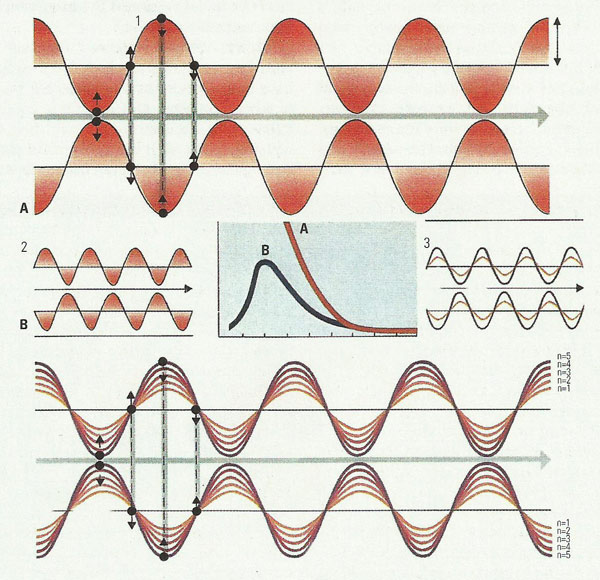A

# quantum mechanicsNineteenth-century physics failed to explain certain observed phenomena – notably, the wavelength-spectrum of radiation emitted by a hot object. In the graph, curve A is the spectrum of radiation (intensities at different wavelengths) that according to theory should have been given off at a temperature of about 1,400°C (2,550°F) (the horizontal scale is in units of 10-4cm). Curve B is the spectrum actually observed. Note that the theoretical spectrum is completely unrealistic, predicting infinite intensity at the shortest wavelengths – a prediction known as the "ultraviolet catastrophe". The 19th-century theory was based on the idea that the radiation was emitted by vibrating, electrically polarized entities – dipoles (1) – whose energy and frequency of vibration could in principle have any magnitude. The difficulty was resolved, around 1900, by Planck's proposal (B3) that each dipole could vibrate only at certain energies, related to its frequency (ν) by the formula E=nhν, where n is 1, 2, 3 etc. and h is a universal constant; a higher-frequency vibration (3) would thus have a higher minimum energy.

 "If somebody says that he can think about quantum physics without becoming dizzy, that shows only that he has not understood anything whatever about it." – Niels Bohr

Quantum mechanics is the science and mathematics that describes the behavior of nature at the atomic and subatomic level. At the heart of quantum mechanics are two basic concepts: 1) that every small bit of matter or energy can behave as if were either a particle or a wave (see wave-particle duality); and 2) that certain combinations of properties such as position and velocity, and energy and time, can't be known with arbitrary precision. The latter idea is encapsulated in Heisenberg's uncertainty principle.

Quantum mechanics was developed during the twentieth century when it became clear that the existing laws of classical mechanics and electromagnetic theory were not successfully applicable to such systems. Because quantum mechanics treats physical events that we cannot directly perceive, it has many concepts unknown in everyday experience. Louis de Broglie struck out from the old quantum theory (see Max Planck and the origins of quantum theory) when he suggested that particles have a wavelike nature (see de Broglie and matter waves). This wavelike nature is significant only for very small particles such as electrons. These ideas were developed by Erwin Schrödinger and others into the branch of quantum mechanics called wave mechanics. Werner Heisenberg worked along parallel lines with a theory incorporating only observable quantities such as energy, using matrix algebra techniques and called matrix mechanics. Paul Dirac incorporated relativistic ideas into quantum mechanics. Quantum electrodynamics was developed by the US physicist Richard Feynman in the 1940s.

## Quantum cosmology

Quantum cosmology is the study of the earliest moments of the universe after the Big Bang, a stage known as the Planck era. Because this field demands an understanding of how spacetime behaves at the quantum level, and there is not yet an adequate quantum theory of gravity, it is highly speculative.

## Quantum dimensions

Quantum dimensions are hypothetical dimensions of spacetime that convert force particles to matter particles and vice versa. Such dimensions are predicted in theories with supersymmetry or superstrings. The pair of particles that are converted into each other are called superpartners.

## Quantum field theory

A quantum field theory is any physical theory that encapsulates both quantum mechanics and special relativity. The fundamental objects in a quantum field theory (QFT) are point-like particles such as electrons. The quintessential example of a quantum field theory (QFT) is quantum electrodynamics (QED). The Standard Model of particle physics is also a QFT.